Get instant live expert help with Excel or Google Sheets“My Excelchat expert helped me in less than 20 minutes, saving me what would have been 5 hours of work!”

#### Post your problem and you’ll get expert help in seconds.

Your message must be at least 40 characters
Our professional experts are available now. Your privacy is guaranteed.

# How to Use the Excel MINA FunctionRead time: 10 minutes

We can use the excel MINA Function to get the LEAST VALUE in a range of cells. The values could be numbers, text, and logical text like TRUE or FALSE. TRUE is read as 1 and FALSE is read as 0 by excel. Text values are read as 0. The steps below will walk through the process.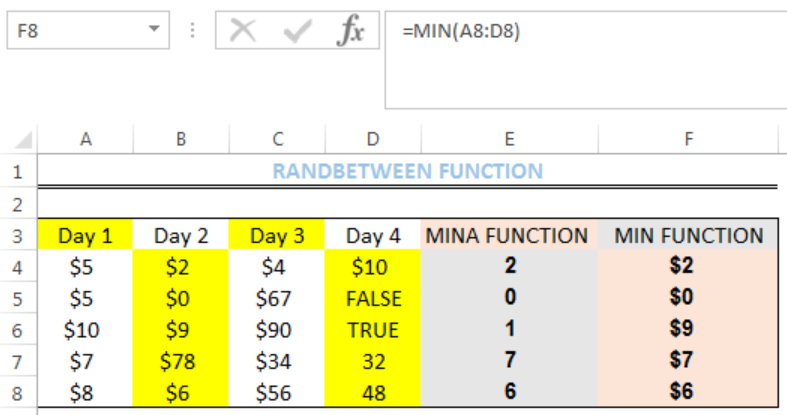Figure 1: How to Use the Excel MINA Function

## General Formula

`=MINA(RANGE)`

## Formula

`=MINA(A4:D4)`

## Setting up the Data

• We will set up the data by inputting the AMOUNT REALISED from FIVE PERSONS for a donation in four days into RANGE A4:D8
• Column E is where we want the formula to return the result for the MINA function
• Column F is where we want the formula to return the result for the MIN function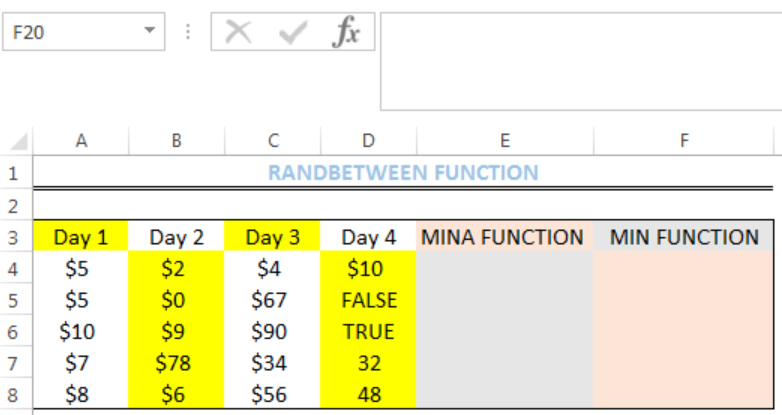Figure 2: Setting up the Data

## Excel MINA Function

• We will click on Cell E4
• We will insert the formula below into the cell
`=MINA(A4:D4)`
• We will press the enter key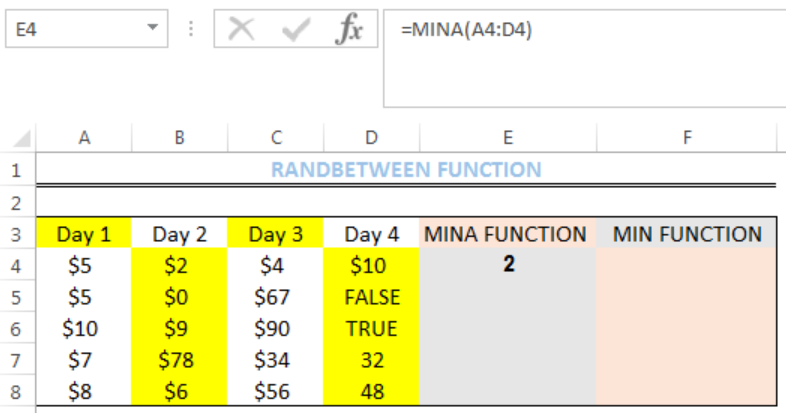Figure 3: Result of the MINA function for range A4:D4

• We will click on Cell E4 again
• We will double-click on the fill handle (the small plus sign at the bottom right of Cell E4) and drag down to copy the formula into the other cells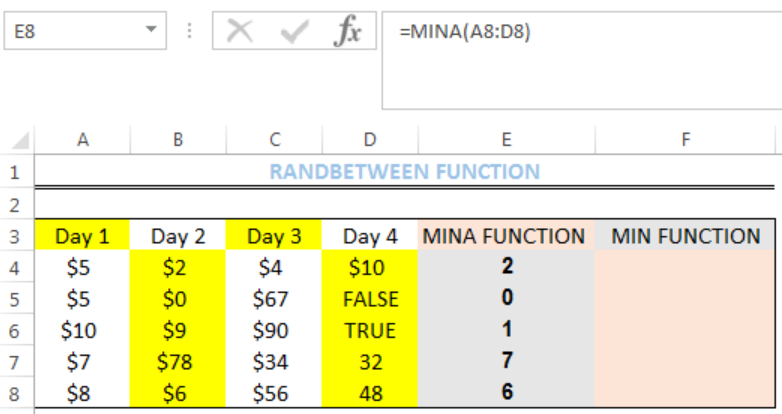Figure 4: Result of the MINA function

## Excel MIN Function

Unlike the EXCEL MINA FUNCTION, the MIN FUNCTION returns only numbers.

• We will click on Cell F4
• We will insert the formula below into the cell
`=MIN(A4:D4)`
• We will press the enter key
• We will click on Cell F4 again
• We will double-click on the fill handle (the small plus sign at the bottom right of Cell F4) and drag down to copy the formula into the other cells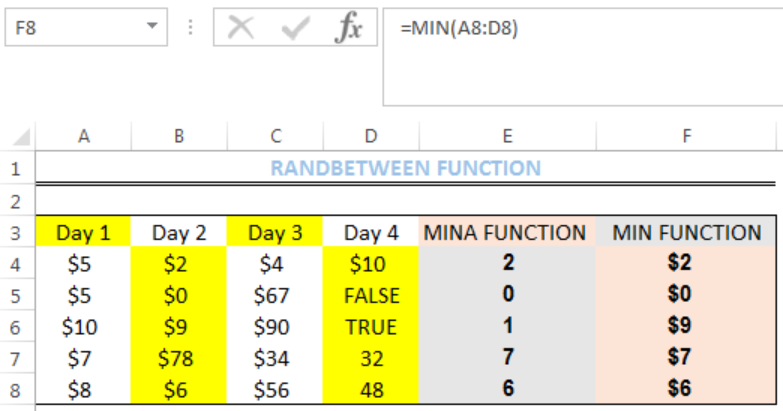Figure 5: Result of the MIN function

## Explanation

The Excel MINA function checks the range for the lowest value, including logical text and text values and returns the least value. If arguments contain no numbers, the Excel MINA function returns 0. It also ignores empty cells.

## Note

The Excel MINA function accepts up to 255 arguments.

## Instant Connection to an Expert through our Excelchat Service

Most of the time, the problem you will need to solve will be more complex than a simple application of a formula or function. If you want to save hours of research and frustration, try our live Excelchat service! Our Excel Experts are available 24/7 to answer any Excel question you may have. We guarantee a connection within 30 seconds and a customized solution within 20 minutes.

Solution examplesI need to count number of dates from a timestamped date in column from the completed date column to show the number of days. I have not successfully been able to do this. I am a novice Excel user. Can you help?
Solved by O. B. in 15 minsUse the Vlookup Function to complete the "employee" column of table 2. Use "job Id" from table 2 as your lookup_value(s) and table 1 as your reference.
Solved by C. H. in 16 minsIf a cell in another sheet is populated I need a vlookup done. If the cell is not populated I need the cell to return blank.
Solved by T. D. in 60 minsI am trying to make a chart that turns a week range red if nothing is entered in the range. If something is entered then I would like it to turn green. Please Help
Solved by E. U. in 43 minsI need a check box to show/hide an answer of an if function
Solved by Z. U. in 23 mins